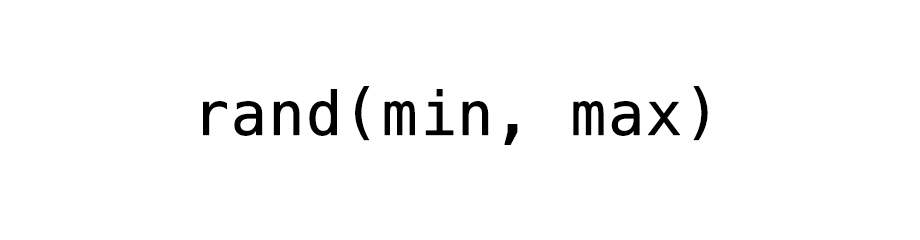# PHP rand( ) function in JavaScript to generate random integers

Reference JavaScriptIn this tutorial we will create a PHP `rand( )` function equivalent in JavaScript to generate random integers.

The `rand()` function generate a random integer.

This function takes two optional integer arguments min and max.

The value of min and max can be between 0 to Number.MAX_SAFE_INTEGER.

Value of `Number.MAX_SAFE_INTEGER` = .

If no arguments is provided then min is set to 0 and max is set to `Number.MAX_SAFE_INTEGER`.

## The rand( ) code

``````function rand(min, max) {
var min = min || 0,
max = max || Number.MAX_SAFE_INTEGER;

return Math.floor(Math.random() * (max - min + 1)) + min;
}
``````

Caution! Values generated by this `rand()` function is not cryptographically secure. So, do not use it for cryptographic purposes.

## Get random integer without passing any argument

The following code will give us a random integer between 0 to Number.MAX_SAFE_INTEGER, both included.

``````function rand(min, max) {
var min = min || 0,
max = max || Number.MAX_SAFE_INTEGER;

return Math.floor(Math.random() * (max - min + 1)) + min;
}

var randomInteger = rand();
``````

## Get random integer with minimum value

The following code will give us a random integer between 10 to Number.MAX_SAFE_INTEGER, both included.

``````function rand(min, max) {
var min = min || 0,
max = max || Number.MAX_SAFE_INTEGER;

return Math.floor(Math.random() * (max - min + 1)) + min;
}

var randomInteger = rand(10);
``````

## Get random integer between min and max value

The following code will give us a random integer between 1 to 10, both included.

``````function rand(min, max) {
var min = min || 0,
max = max || Number.MAX_SAFE_INTEGER;

return Math.floor(Math.random() * (max - min + 1)) + min;
}

var randomInteger = rand(1, 10);
``````

If min and max are equal then `rand()` will return the same number.

Following code will give us 5 as both min and max is set to 5.

``````function rand(min, max) {
var min = min || 0,
max = max || Number.MAX_SAFE_INTEGER;

return Math.floor(Math.random() * (max - min + 1)) + min;
}

var randomInteger = rand(5, 5);
``````

## rand( ) code with checks

The following code makes sure that min and max are between 0 and Number.MAX_SAFE_INTEGER.

``````function rand(min, max) {
var min = min || 0,
max = max || Number.MAX_SAFE_INTEGER;

if (min < 0) {
min = 0;
}
else if (min > Number.MAX_SAFE_INTEGER) {
min = Number.MAX_SAFE_INTEGER
}

if (max < 0) {
max = 0;
}
else if (max > Number.MAX_SAFE_INTEGER) {
max = Number.MAX_SAFE_INTEGER
}

return Math.floor(Math.random() * (max - min + 1)) + min;
}
``````